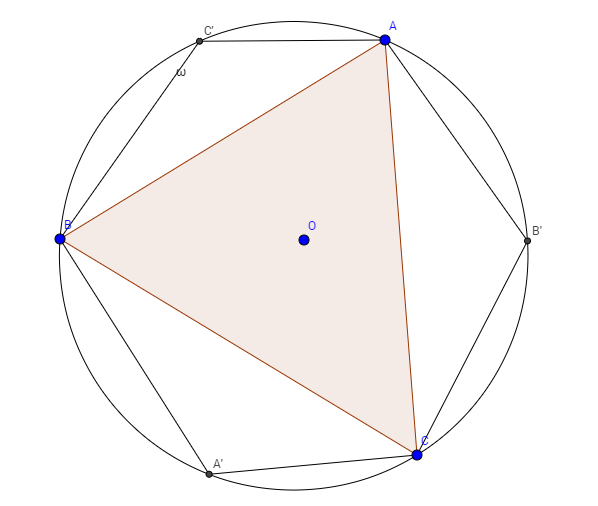# A Triangle Evolves Into A Hexagon

Geometry Level 2Consider an acute angled $\Delta ABC$ with circumcircle $\omega$ and circumcenter $O$. Now, construct points (on the circumcircle) diametrically opposite to the vertices of the triangle, and name them $A', B'$ and $C'$ as shown in the figure. The area of $\Delta ABC$ is $18$ square units.

Find the area of polygon $AB'CA'BC'$.

×

Problem Loading...

Note Loading...

Set Loading...